# Bihar Board Class 10th Maths Solutions Chapter 10 Circles Ex 10.2

Bihar Board Class 10th Maths Solutions Chapter 10 Circles Ex 10.2 Textbook Questions and Answers.

## BSEB Bihar Board Class 10th Maths Solutions Chapter 10 Circles Ex 10.2Question 1.
From a point Q, the length of the tangent to a circle is 24 cm and the distance of Q from the centre is 25 cm. The radius of the circle is
(A) 7 cm
(B) 12 cm
(C) 15 cm
Solution:
Since QT is a tangent to the circle at T and OT is the radius.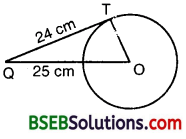∴ OT ⊥ QT
It is given that OQ = 25 cm and QT = 24 cm.
By Pythagoras theorem, we have :
OQ² = QT² + OT²
or OT² = OQ² – QT²
or OT² = 25² – 24²
= (25 + 24)(25 – 24)
= 49 x 1 = 49
or OT = $$\sqrt{49}$$
= 7
Hence, radius of the circle is 7 cm, i.e., (A)

Question 2.
In figure, if.TP and TQ are the two tangents to a circle with centre O so that ∠POQ = 110°, then ∠PTQ is equal to
(A) 60°
(B) 70°
(C) 80°
(D) 90°
Solution:
Since TP and TQ are tangents to a circle with centre Oso that ∠POQ = 110°, therefore
OP ⊥ PT and OQ ⊥ QT.
So, ∠OPT = 90° and ∠OQT = 90°.
In the quadrilateral TPOQ, we have :
∠PTQ + ∠TPO + ∠POQ + ∠OQT = 360°
So, ∠PTQ + 90° + 110° + 90° = 360°
or ∠PTQ + 290° = 360°
or ∠PTQ = 360° – 290° = 70°
i.e., (B).Question 3.
If tangents PA and PB from a point P to a circle with centre O are inclined to each other at angle of 80°, then ∠POA is equal to
(A) 50°
(B) 60°
Solution:
Since PA and PB are tangents to a circle with centre 0, thereforeOA ⊥ AP and OB ⊥ BP.
So, ∠OAP = 90° and ∠OBP = 90°.
In the quadrilateral PAOB, we have :
∠APB + ∠PAO + ∠AOB + ∠OBP = 360°
or 80° + 90° + ∠AOB + 90° = 360°
or 260° + ∠AOB = 360°
or ∠AOB = 360° – 260° = 100°
In the right As OAP and OBP, we have :
OP = OP [Common]
∠OAP = ∠OBP [Each = 90°]
∆ OAP ≅ ∆ OBP (RHS congruence rule)
So, ∠POA = ∠POB [C.P.C.T.]
∠POA = $$\frac { 1 }{ 2 }$$∠AOB = $$\frac { 1 }{ 2 }$$ x 100° = 50°,
i.e., (A).

Question 4.
Prove that the tangents drawn at the ends of a diameter of a circle are parallel.
Solution:
Let PQ be a diameter of the given circle with centre O.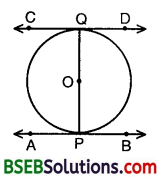Let AB and CD be the tangents drawn to the circle at the end points of the diameter PQ respectively.
Since tangent at a point to a circle is perpendicular to the radius through the point, therefore
PQ ⊥ AB and PQ ⊥ CD
So, ∠APQ = ∠PQD
or AB || CD [∵∠APQ and ∠PQD are alternate angles]

Question 5.
Prove that the perpendicular at the point of contact to the tangent to a circle passes through the centre.
Solution:
Let AB be the tangent drawn at the point P on the circle with centre 0.If possible, let PQ be perpendicular to AB, not passing through O.
Join OP.
Since tangent at a point to a circle is perpendicular to the radius through the point, therefore
AB ⊥ OP, i.e., ∠OPB = 90°
Also, ∠QPB = 90° (construction)
∴ ∠QPB = ∠OPB, which is not possible as a part cannot be equal to whole.
Hence, the perpendicular at the point of contact to the tangent to a circle passes through the centre.

Question 6.
The length of a tangent from a point A at distance 5 cm from the centre of the circle is 4 cm. Find the radius of the circle.
Solution:
Since tangent to a circle is perpendicular to the radius through the point of contact, therefore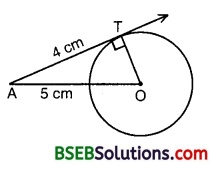∠OTA = 90°
In right ∆ OTA, we have :
OA² = OT² + AT²
5² = OT² + 4²
OT² = 5² – 4² = 25 – 16 = 9
OT = $$\sqrt{9}$$ = 3
Hence, radius of the circle is 3 cm.Question 7.
Two concentric circles are of radii 5 cm and 3 cm. Find the length of the chord of the larger circle which touches the smaller circle.
Solution:
Let O be the common centre of two concentric circles, and let AB be a chord of the larger circle touching the smaller circle at P.Join OP.
Since OP is the radius of the smaller circle and AB is tangent to this circle at P, therefore
OP ⊥ AB.
We know that the perpendicular drawn from the centre of a circle to any chord of the circle bisects the chord.
So, OP ⊥ AB and AP = BP.
In right ∆ APO, we have :
OA² = AP² + OP²
or 5² = AP² + 3²
or AP² = 5² – 3²
= 25 – 9 = 16
or AP = $$\sqrt{16}$$ = 4
Now, AB = 2AP [∵ AP = BP]
= 2 x 4 = 8
Hence, the length of the chord of the larger circle which touches the smaller circle is 8 cm.

Question 8.
A quadrilateral ABCD is drawn to circumscribe a circle (See figure).
Prove that AB + CD = AD + BC.Solution:
Let the quadrilateral ABCD be drawn to circumscribe a circle as shown in the figure.
i.e., the circle touches the sides AB, BC, CD and DA at P,Q, R and S respectively.
Since lengths of two tangents drawn from an external point to a circle are equal, therefore
AP = AS
BP = BQ
DR = DS
CR = CQ
(AP + BP) + (CR + RD) = (BQ + QC) + (DS + SA)
or AB + CD = BC + DA
which proves the result.

Question 9.
In the figure, XY and X’Y’ are two parallel tangents to a circle with centre O and another tangent AB with point of contact C intersecting XY at A and X’Y’ at B. Prove that ∠AOB = 90°.Solution:
Since tangents drawn from an external point to a circle are equal, therefore
AP = AC. Thus, in As PAO and CAO, we have :
AP = AC
AO – AO [Common]
and, PO = OC[Radii of the same circle]
By SSS criterion of congruence, we have :
∆ PAO ≅ ∆ CAO .
or ∠PAO = ∠CAO
So, ∠PAC = 2∠CAO
Similarly, we can prove that
∠QBO = ∠CBO
So, ∠CBQ = 2∠CBO
Now, ∠PAC + ∠CBQ = 180° [Sum of the interior angles on the same side of transversal is 180°]
or 2∠CAO + 2∠CBO = 180° or ∠CAO + ∠CBO = 90°
But in ∆ AOB,
∠CAO + ∠CBO + ∠AOB = 180° [By angle sum property of a triangle]
So, 180° – ∠AOB = 90°
Hence, ∠AOB = 90°Question 10.
Prove that the angle between the two tangents drawn from an external point to a circle is supplementary to the angle subtended by the line segment joining the points of contact at the centre.
[Annual Paper (Outside Delhi) 2008]
Solution:
Let PA and PB be two tangents drawn from an external point P to a circle with centre O.
We have to prove that∠AOB + ∠APB = 180°
In right As OAP and OBP, we have : B
PA = PB [Tangents drawn from an external point are equal]
OA = OB [Radii of the same circle]
and, OP = OP [Common]
∴ By SSS criterion of congruence
∆ AOP ≅ ∆ BOP.
So, ∠OPA = ∠OPB
and, ∠AOP = ∠BOP So, ∠APB = 2∠OPA
and, ∠AOB = 2∠AOP
But, ∠AOP = 90° – ∠OPA[ ∵ ∆ OAP is right triangle]
∴ 2∠AOP = 180° – 2∠OPA
or ∠AOB = 180° – ∠APB
or ∠AOB + ∠APB = 180°
∠A + ∠APB + ∠B + ∠AOB = 360° … (1)
[Angle sum property of a quadrilateral]
Also, ∠A = 90° and ∠B = 90° … (2)
So, 90° + ∠APB + 90° + ∠AOB = 3G0° [From (1) and (2)]
Hence, ∠APB + ∠AOB – 360° – 90° – 90° = 180°

Question 11.
Prove that the parallelogram circumscribing a circle is a rhombus.
Solution:
Let ABCD be a parallelogram such that its sides touch a circle .
We know that the tangents to a circle from an exterior point are equal in length.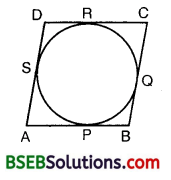∴ AP = AS
BP = BQ
CR = CQ
and, DR = DS
(AP + BP) + (CR + DR) = (AS + DS) + (BQ + CQ)
So, AB + CD = AD + BC
or 2AB = 2BC
[∵ABCD is a ||gm ∴AB = CD and BC = AD]
or AB = BC
Thus, AB = BC = CD = AD.
Hence, ABCD is a rhombus.Question 12.
A triangle ABC is drawn to circumscribe a circle of radius 4 cm such that the segments BD and DC into which BC is divided by the point of contact D are of lengths 8 cm and 6 cm respectively (See figure). Find the sides AB and AC.Solution:
Let the ∆ ABC be drawn to centre O and radius 4 cm.
i.e., the circle touches the sides BC, CA and AB at D, E and F respectively.
It is given that BD = 8 cm and CD = 6 cm.Since lengths of two tangents drawn from an external point to a circle are equal, therefore
BF = BD = 8 cm, CE = CD = 6 cm and let AF = AE = x cm.
Then, the sides of the triangle are 14 cm, (x + 6) cm and (x+8)cm.
2s = 14 + (x + 6) + (x + 8)
= 28 + 2x or s = 14 + x
s – a – 14 + x – 14 = x,
s – b – 14 + x – x – 6 = 8
and s – c = 14 + x – x – 8 = 6
∴ Area (∆ ABC) = $$\sqrt{s(s-a)(s-b)(s-c)}$$
= $$\sqrt{(14+x)(x)(8)(6)}$$
= $$\sqrt{48\left(x^{2}+14 x\right)}$$
Also, Area (A ABC)
= Area (∆ OBC) + Area (∆ OCA) + Area (∆ OAB)
= $$\frac { 1 }{ 2 }$$ x BC x OD + $$\frac { 1 }{ 2 }$$ x CA x OE + $$\frac { 1 }{ 2 }$$ x AB x OF
= $$\frac { 1 }{ 2 }$$ x 14 x 4 + $$\frac { 1 }{ 2 }$$ x(x + 6) x 4 + $$\frac { 1 }{ 2 }$$ x (x + 8) x 4
= 2(14 + x – + 6 + x + 8) = 2(28 + 2x)
∴ $$\sqrt{48\left(x^{2}+14 x\right)}$$ = 2(28 + 2x) = 4(14 + x)
Squaring, we get
48(x² + 14x) = 16(14 + x)²
or 3(x² + 14x) = 196 + 28x + x²
or 2x³ + 14x 196 = 0 or x² + 7x – 98 = 0
(x – 7)(x + 14) = 0 i.e., x = 7 or x = – 14
But x cannot be negative.
∴ x = 7
Hence, the sides AB and AC are 15 cm and 13 cm respectively.Question 13.
Prove that opposite sides of a quadrilateral circumscribing a circle subtend supplementary angles at the centre of the circle.
Solution:
Let a circle with centre O touches the sides AB, BC, CD and DA of a quadrilateral ABCD at the points P, Q, R and S respectively.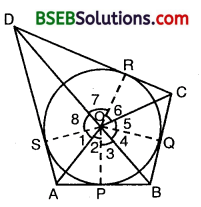We are to prove that
∠AOB + ∠COD = 180°
and ∠AOD + ∠BOC = 180°
Join OP, OQ, OR and OS.
Since the two tangents drawn from an external point to a circle subtend equal angles at the centre, therefore
∠1 = ∠2, ∠3 = ∠4, ∠5 = ∠6 and ∠7 = ∠8.
Now, ∠1 + ∠2 + ∠3 + ∠4 + ∠5 + ∠6 + ∠7 + ∠8 = 360°
[∵ Sum of all the angles around a point is 360°] So, 2(∠2 + ∠3 + ∠6 + ∠7) = 360°
and 2(∠1 + ∠8 + ∠4 + ∠5) = 360°
So, (∠2 + ∠3) + (∠6 + ∠7) = 180°
and (∠1 + ∠8) + (∠4 + ∠5) = 180°
So, ∠AOB + ∠COD = 180°
[∵ ∠2 + ∠3 = ∠AOB, ∠6+ ∠7 = ∠COD ∠1 + ∠8 = ∠AOD and ∠4 + ∠5 = ∠BOC]
and ∠AOD + ∠BOC = 180°